V1

2022/12/06阅读：80主题：萌绿

# JS逆向系列-猿人学web第一题

• 浏览器安装`Cookie AutoDelete`插件，便于清除cookie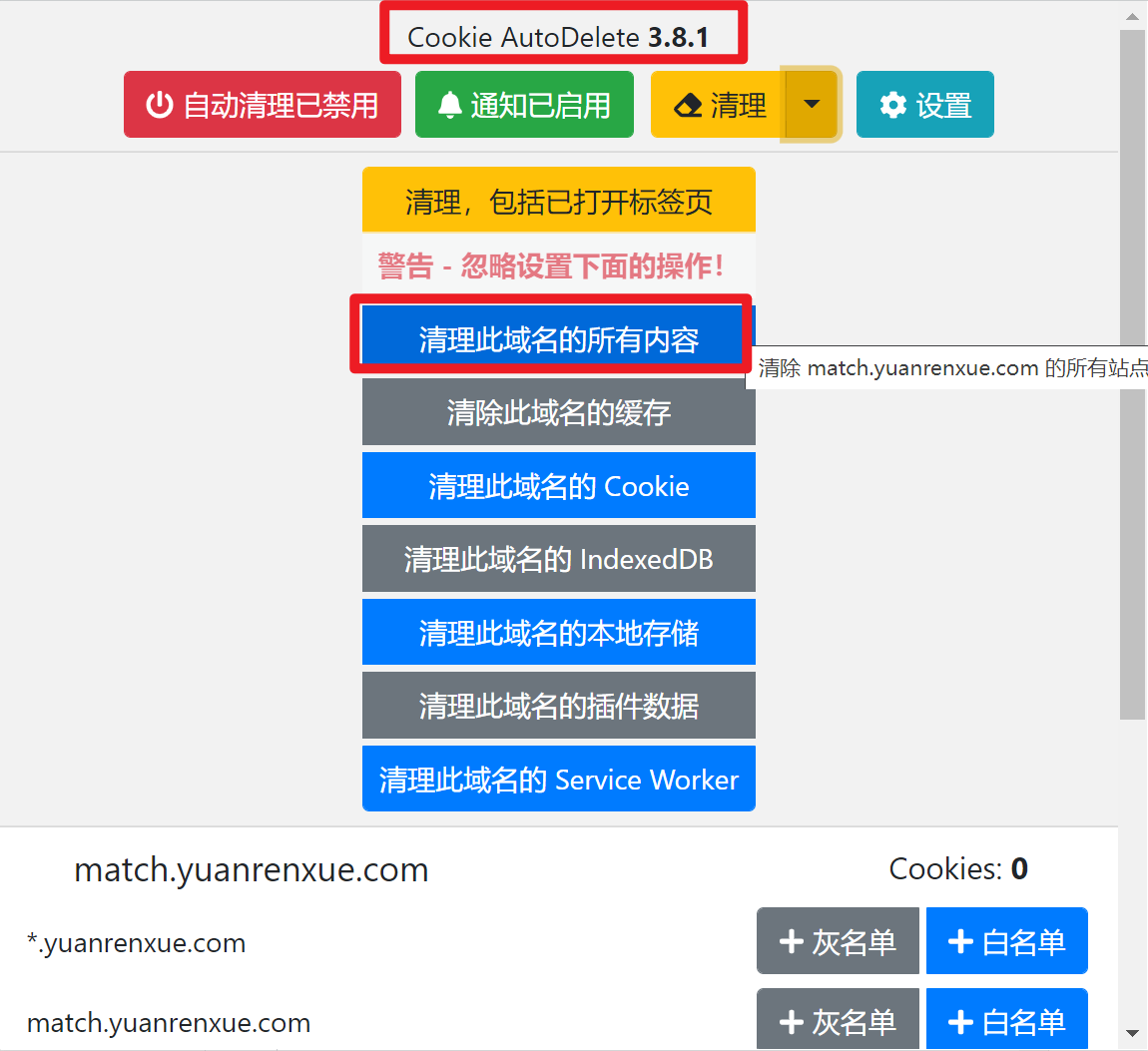(关注本公众号(程序员小木屋)回复"cookie"可获取下载地址)
• 安装好python，安装好nodejs，安装好pycahrm，安装好requests包，安装好execjs
1. 打开网址`https://match.yuanrenxue.com/match/1#`，并打开开发者工具。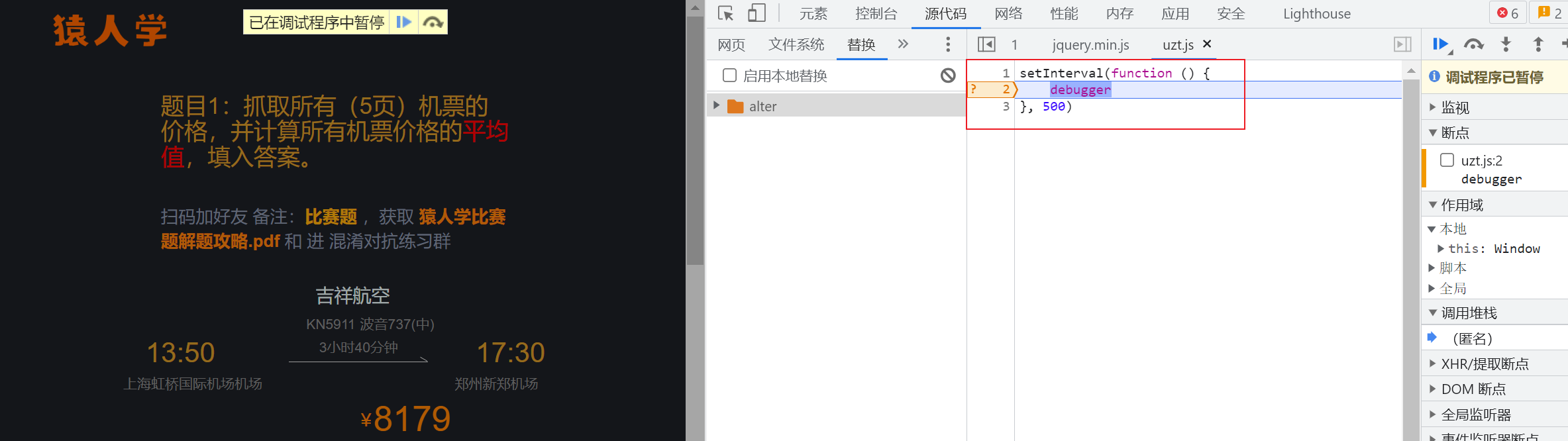首先要解决的是无限`debugger`问题，上述代码的意思是，有一个每隔500毫秒就运行一次的函数，函数的内容就是在此处暂停，我们需要解决的是，不让函数在此处暂停。

1. `网络`tab栏查看所有接口，找到传输机票价格的接口经过比较，此接口是返回价格的接口

1. 查看接口传参，检查接口是否有加密参数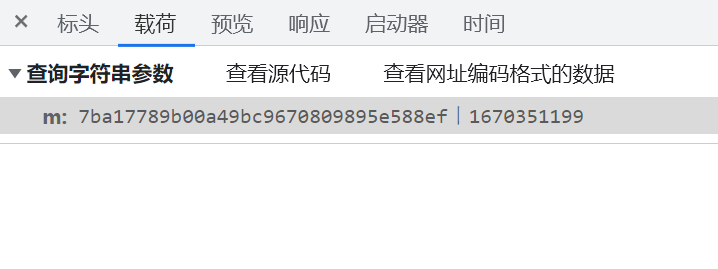经过查看，headers中没有异常参数，但是传参中有一个参数`m`，我们需要找到这个`m`是如何生成的。

1. 点击`启动器`tab页，查看调用堆栈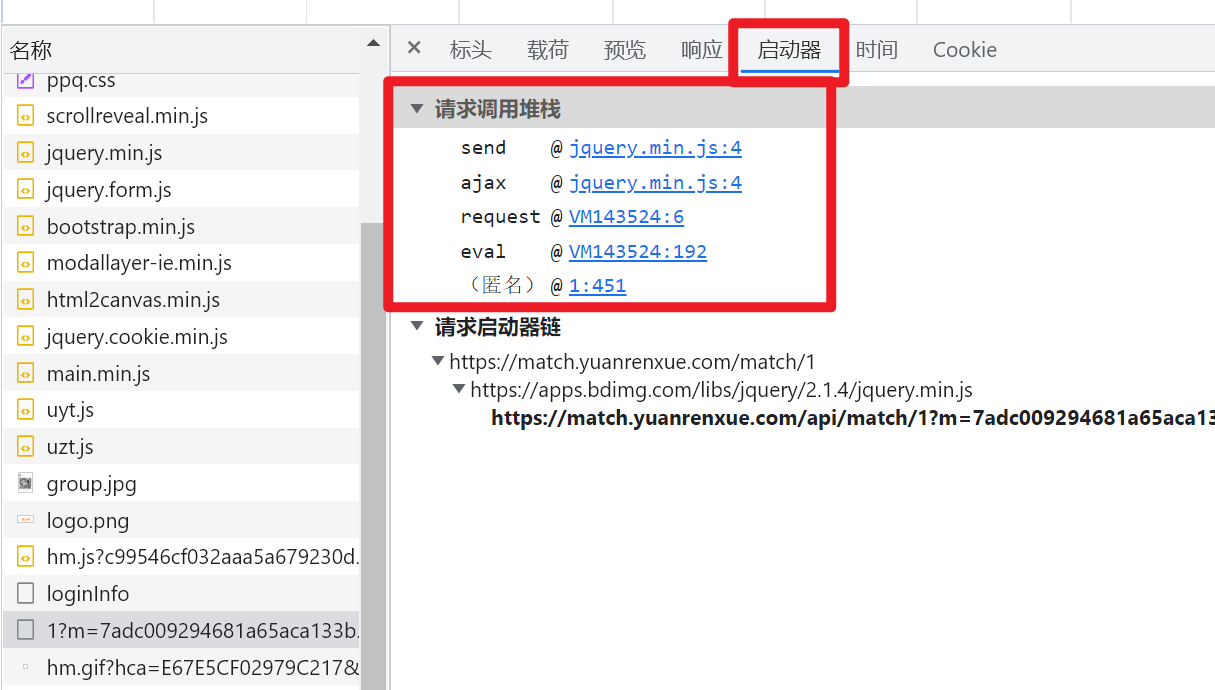`调用堆栈`是指此接口执行的代码顺序

2. 依次点击进入堆栈，并在能打断点的地方打上断点

• 进入js文件后，通过点击左下角的`{}`进行格式化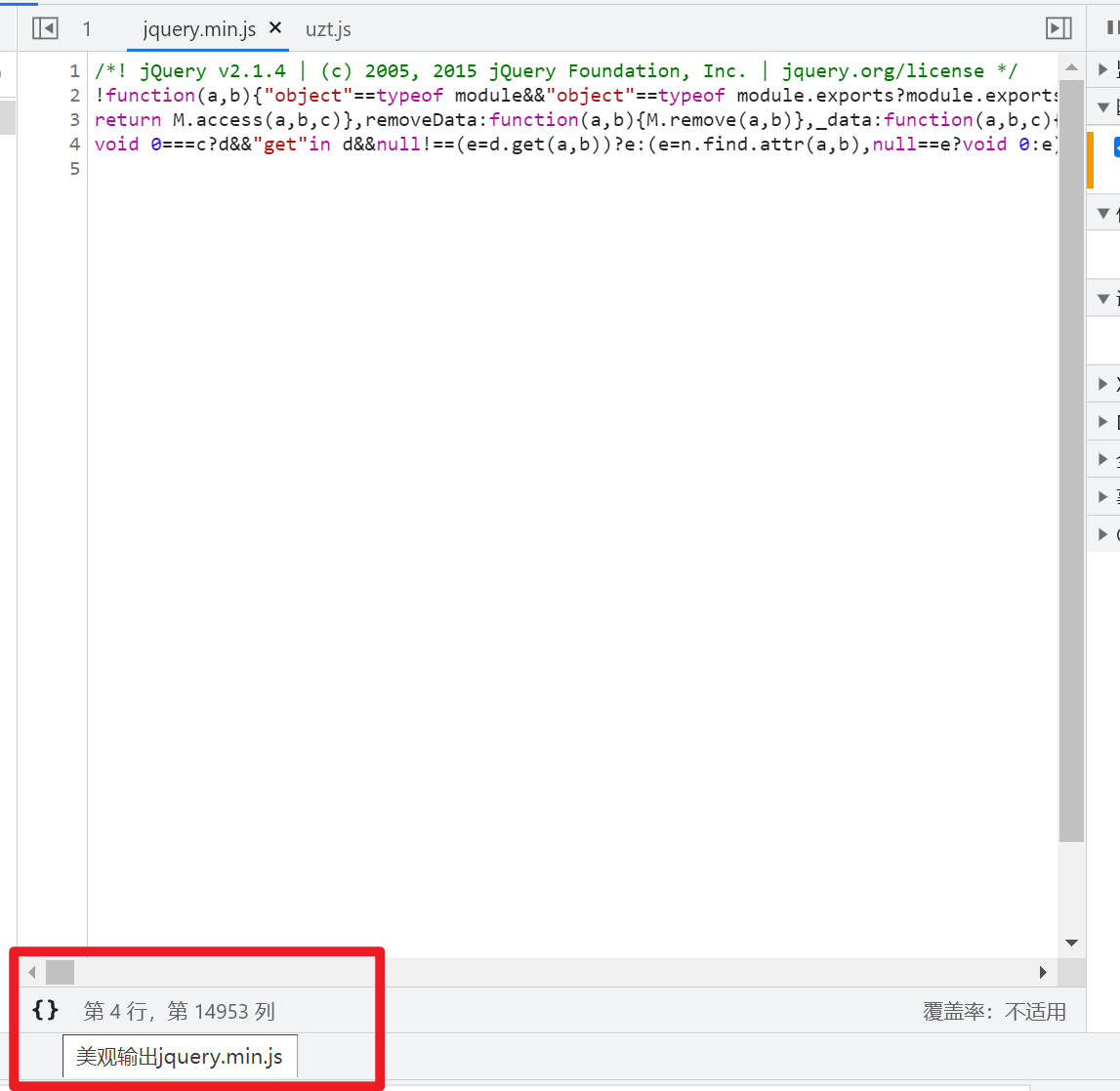• 找到对应的行后，点击左侧的行号就能打断点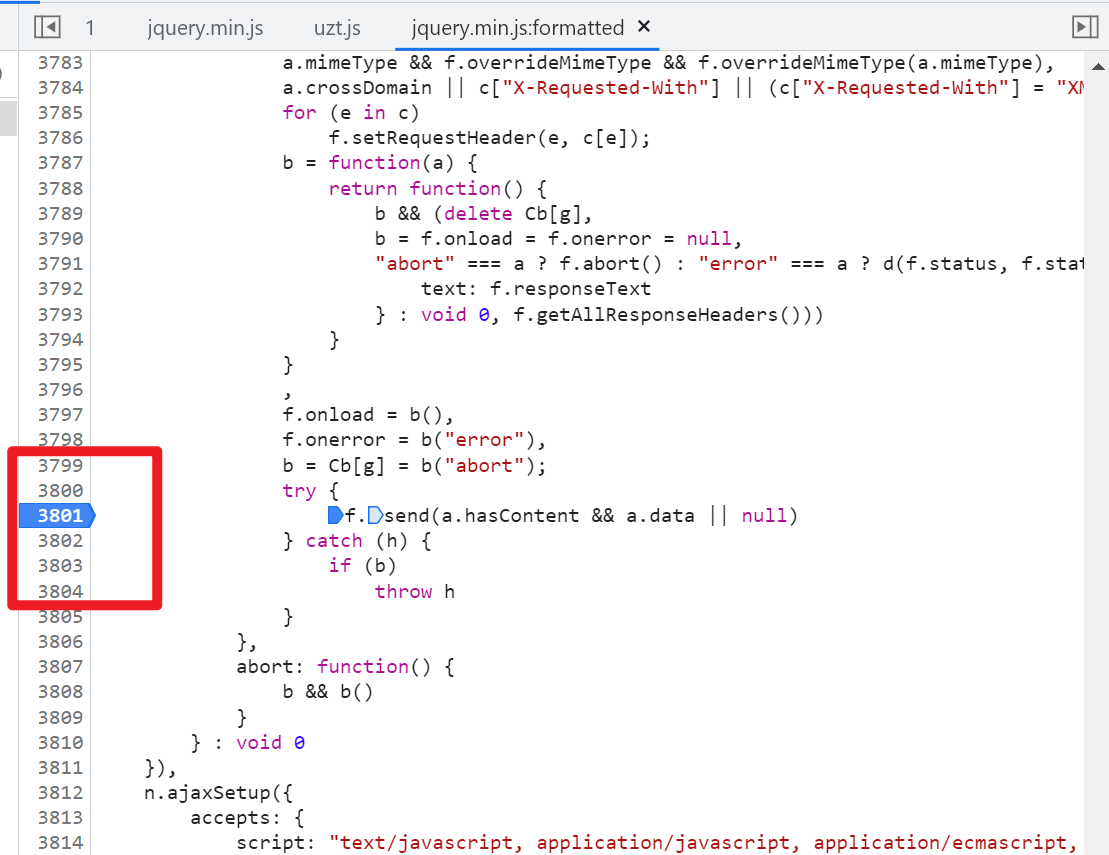1. 使用插件清除cookie后，重新刷新界面，查看断点位置的数据，找到第一次生成`m`参数的位置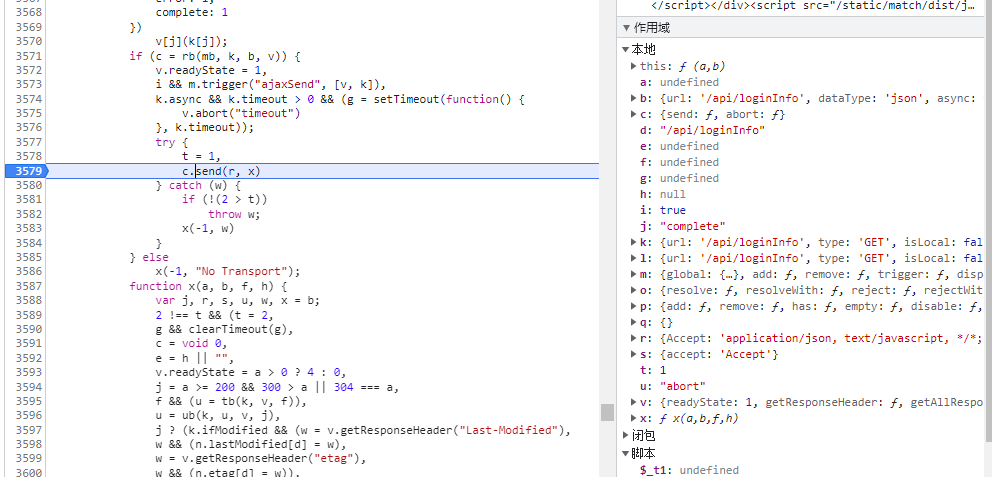`c.send`位置没有`m`参数，继续执行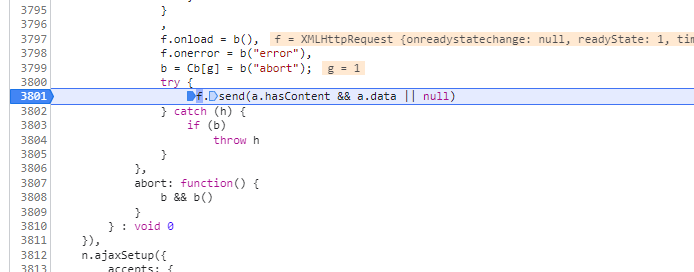`f.send`也没有看到，继续执行这一长串这也没看到，继续执行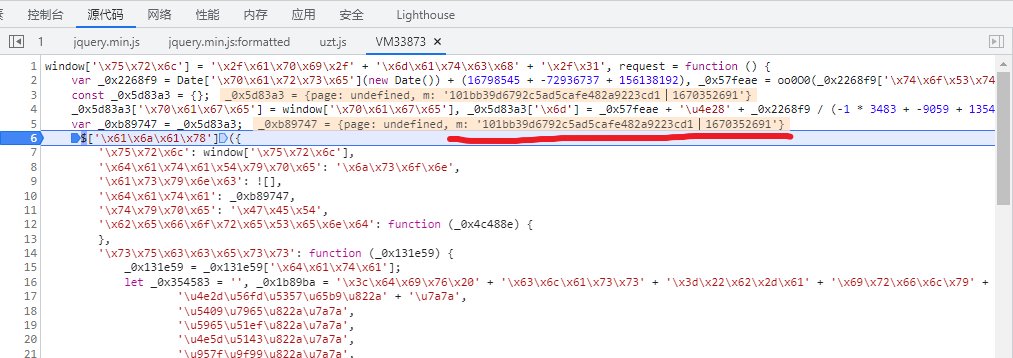欸，最后终于看到`m`了！

1. 观察此处的代码，分析`m`是如何生成的

• 此处的代码一看就进行了混淆，我们可以逐个拆分，输出到控制台中，查看每个变量都是什么含义。
``_0x5d83a3['\x70\x61\x67\x65'] = window['\x70\x61\x67\x65'], _0x5d83a3['\x6d'] = _0x57feae + '\u4e28' + _0x2268f9 / (-1 * 3483 + -9059 + 13542);``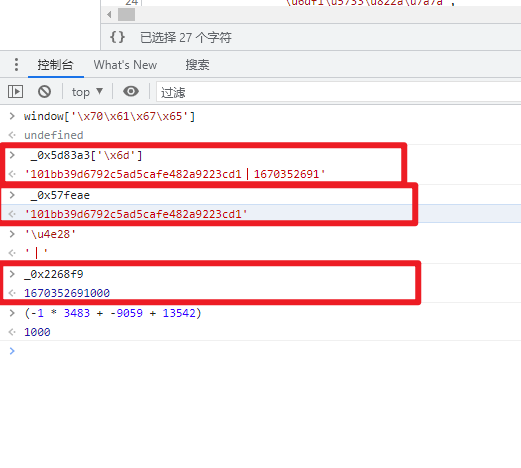看到了没有，`_0x5d83a3['\x6d']`就是`m``_0x57feae``m`的前半部分，`_0x2268f9 * 1000`就是`m`的后半部分(即时间戳)。

1. 现在得到了`m`的后半部分，继续查找前半部分，看第2行，前半部分是在这里生成的
• 因为程序已经执行到了第6行，我们现在查看第2行的结果，可能已经被污染了，所以我们选择在第2行打断点，重新执行，让程序变为第2行还未执行的状态。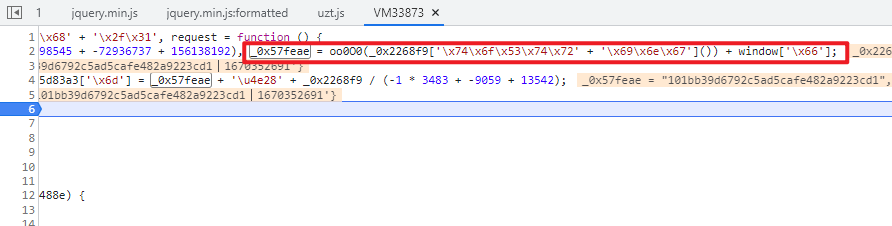我们依次执行第二行的函数，如下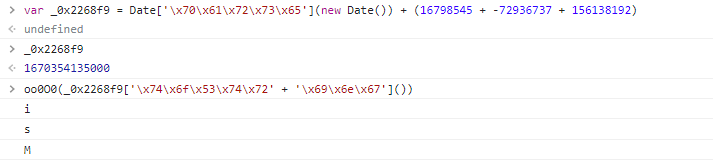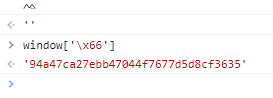发现`_0x2268f9`是时间戳，`oo0O0(_0x2268f9['\x74\x6f\x53\x74\x72' + '\x69\x6e\x67']())`是一个暂时不知道干啥的函数，`window['\x66']`正确输出了`m`的前半段。

1. 寻找`window['\x66']`

`'\x66'``'f'`，我们要找到`window.f`这个变量。 但是根据我的`ctrl+f`查找，并没有找到`window.f`，这是我们大胆假设，难道这个变量是在前面那一段不知道干啥用的函数生成的？我们进入`oo0O0`函数中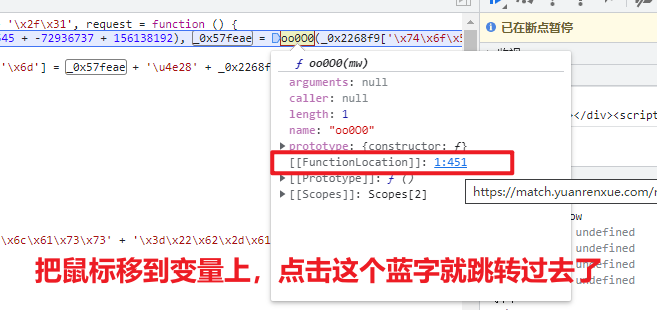1. 解混淆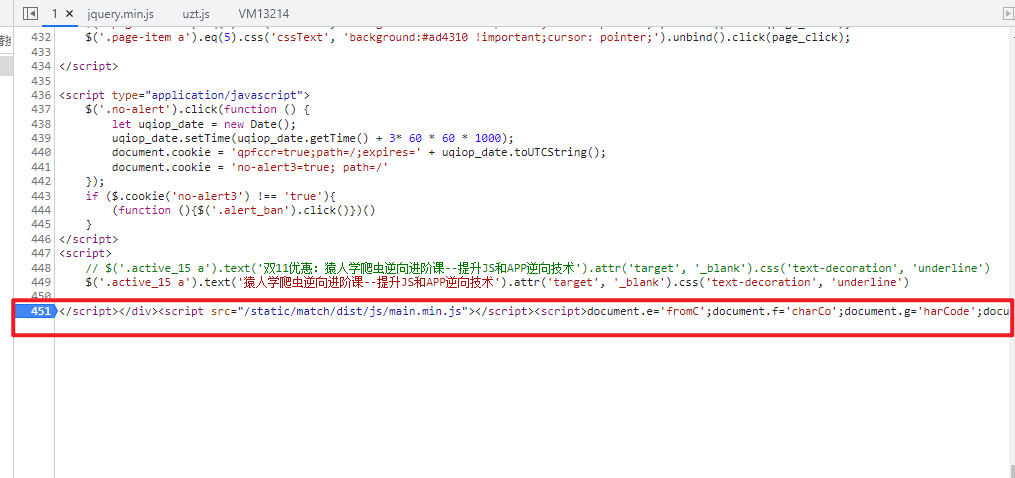代码跳转到了这里，`oo0O0`函数就隐藏在其中，这可怎么办呢？

``function oo0O0(mw) {    window.b = '';    for (var i = 0,    len = window.a.length; i < len; i++) {        console.log(window.a[i]);        window.b += String[document.e + document.g](window.a[i][document.f + document.h]() - i - window.c)    }    var U = ['W5r5W6VdIHZcT8kU', 'WQ8CWRaxWQirAW=='];    var J = function(o, E) {        o = o - 0x0;        var N = U[o];        if (J['bSSGte'] === undefined) {            var Y = function(w) {                var m = 'abcdefghijklmnopqrstuvwxyzABCDEFGHIJKLMNOPQRSTUVWXYZ0123456789+/=',                T = String(w)['replace'](/=+\$/, '');                var A = '';                for (var C = 0x0,                b, W, l = 0x0; W = T['charAt'](l++);~W && (b = C % 0x4 ? b * 0x40 + W: W, C++%0x4) ? A += String['fromCharCode'](0xff & b >> ( - 0x2 * C & 0x6)) : 0x0) {                    W = m['indexOf'](W)                }                return A            };            var t = function(w, m) {                var T = [],                A = 0x0,                C,                b = '',                W = '';                w = Y(w);                for (var R = 0x0,                v = w['length']; R < v; R++) {                    W += '%' + ('00' + w['charCodeAt'](R)['toString'](0x10))['slice']( - 0x2)                }                w = decodeURIComponent(W);                var l;                for (l = 0x0; l < 0x100; l++) {                    T[l] = l                }                for (l = 0x0; l < 0x100; l++) {                    A = (A + T[l] + m['charCodeAt'](l % m['length'])) % 0x100,                    C = T[l],                    T[l] = T[A],                    T[A] = C                }                l = 0x0,                A = 0x0;                for (var L = 0x0; L < w['length']; L++) {                    l = (l + 0x1) % 0x100,                    A = (A + T[l]) % 0x100,                    C = T[l],                    T[l] = T[A],                    T[A] = C,                    b += String['fromCharCode'](w['charCodeAt'](L) ^ T[(T[l] + T[A]) % 0x100])                }                return b            };            J['luAabU'] = t,            J['qlVPZg'] = {},            J['bSSGte'] = !![]        }        var H = J['qlVPZg'][o];        return H === undefined ? (J['TUDBIJ'] === undefined && (J['TUDBIJ'] = !![]), N = J['luAabU'](N, E), J['qlVPZg'][o] = N) : N = H,        N    };    eval(atob(window['b'])[J('0x0', ']dQW')](J('0x1', 'GTu!'), '\x27' + mw + '\x27'));    return ''};``

``eval(atob(window['b'])[J('0x0', ']dQW')](J('0x1', 'GTu!'), '\x27' + mw + '\x27'));``

`J`变量不存在，但是`atob(window['b']`输出了一段代码，看到代码里的`md5`字样，你的心有没有激动起来呀~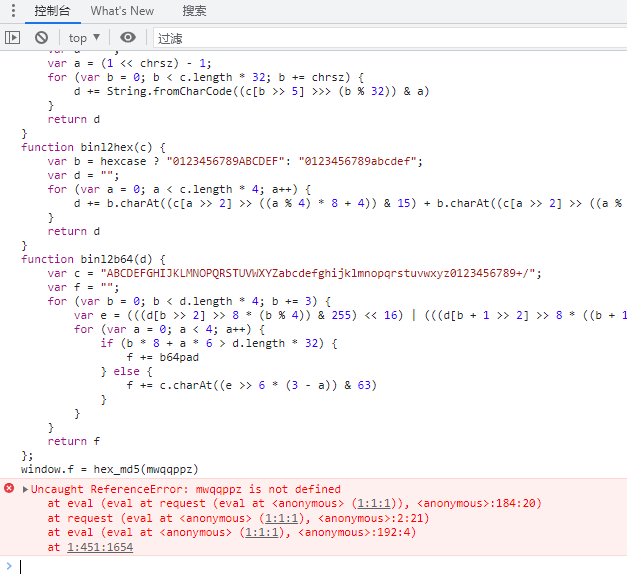咦报错了？不要慌！这里缺少的`mwqqppz`不就是执行`oo0O0`函数时传进来的时间戳嘛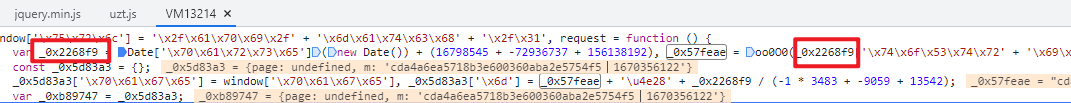重新执行一下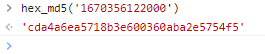出来了！ 跟继续执行的结果比较一下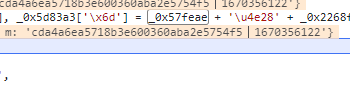一摸一样！11. 太棒了！接下来试验一下

• 创建一个`hex_md5.js`文件，把`atob(window['b']`展示的这段代码复制进去
• 把时间戳的生成方法也搞进去(还记得在哪里吧)，然后定义一个`run`函数去执行它(这里又需要一些js基础)
• 创建一个`run.py`文件，然后输入以下代码，我们先试试能不能运行这个js文件
``import execjsfrom pathlib import Pathcontext = execjs.compile(Path("./hex_md5.js").read_text())[ts, hex_md5] = context.call('run')print(ts, hex_md5)``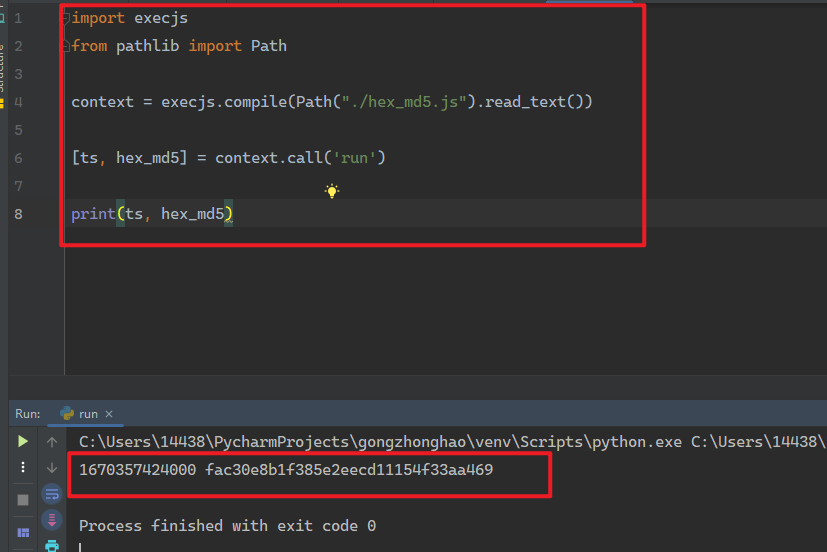可以运行太棒了！

1. 页面查看接口的规律

• 不同页的接口page参数发生了改变，第二页是page=2，第三页是page=3...；
• 四五这两页进行了锁定，需要添加设置 `user-agent='yuanrenxue.project'`
1. python实现

`run.py`中写入如下代码(不懂的地方看注释哦)

``import execjsfrom pathlib import Pathimport requestscontext = execjs.compile(Path("./hex_md5.js").read_text())# url地址url = "https://match.yuanrenxue.com/api/match/1"# 定义headersheaders = {"user-agent": "yuanrenxue.project"}[ts, hex_md5] = context.call("run")# 生成m参数m = f"{hex_md5}丨{ts}"# 记录总和res = 0for page in range(1, 6):    # 生成params    params = {"page": page, "m": m}    # 获取数据    resp = requests.get(url, params=params, headers=headers)    json_data = resp.json()    # {'status': '1', 'state': 'success', 'data': [{'value': 8179}, {'value': 6177},     # {'value': 4174}, {'value': 5945}, {'value': 9556}, {'value': 2318}, {'value': 4},     # {'value': 2653}, {'value': 4855}, {'value': 1370}]}    res += sum(map(lambda x: x["value"], json_data["data"]))print("总和为", res)``

``总和为 235000``
1. 因为我没有注册账号，所以没法提交，但是接口响应都正确，结果肯定没问题啦。

end

V1